### Fractional Numbers - Worksheets

CBSE Worksheet-01
Class - IV Mathematics – Fractional Numbers

Time Allowed: 30 minutes

1. Fill in the blanks with suitable words:
1.  $\frac{3}{4}$ + __ = $\frac{12}{4}$

2. The improper fraction of $4\frac{7}{10}$ is ________.

3. Improper fraction of $4\frac{1}{5}$ is ________.

4. $4\frac{3}{5}+5\frac{2}{5}=$ ________

5. In $\frac{9}{23}$; ________ is denominator.

2. State whether each of the following statement is True or False:
1. The colored part in the below figure in the fraction is $\frac{7}{12}$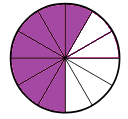2. $\frac{21}{5}=4\frac{1}{5}$

3. $\frac{2}{5}$ and $\frac{7}{5}$ are like fractions.

4. $\frac{1}{2}+\frac{3}{2}+\frac{5}{2}=4$

5. $\frac{5}{2}-\frac{3}{2}-\frac{2}{2}=0$

3. What part of the diagram is the coloured?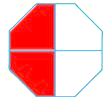1. $\frac{4}{2}$

2. $\frac{3}{2}$

3. $\frac{2}{2}$

4. $\frac{1}{2}$

4. Numerator in the fraction $\frac{5}{7}$ is

1. $\frac{5}{7}$

2. 5

3. 7

4. $\frac{1}{7}$

5. Find the sum: $\frac{1}{10},\frac{3}{10}\phantom{\rule{thinmathspace}{0ex}}\phantom{\rule{thinmathspace}{0ex}}$ and $\frac{4}{10}$

1. $\frac{8}{30}$

2. $\frac{4}{20}$

3. None of these

4. $\frac{8}{10}$

6. Compare the pair of fractions using<,> or=sign. $\frac{4}{8}\phantom{\rule{thinmathspace}{0ex}}?\frac{8}{9}$

1. >

2. <

3. None of these

4. =

7. What is obtained while converting $\frac{16}{5}$ into mixed fraction?

1. $1\frac{4}{5}$

2. $3\frac{1}{5}$

3. $2\frac{3}{5}$

4. $1\frac{3}{5}$

8. Match the following:

 (a)   110.......410$\frac{1}{10}.......\frac{4}{10}$ (i) = (b)   320.......120$\frac{3}{20}.......\frac{1}{20}$ (ii) < (c)    210.......15$\frac{2}{10}.......\frac{1}{5}$ (iii) > (d)   210.......110=310$\frac{2}{10}.......\frac{1}{10}=\frac{3}{10}$ (iv) +
9. Arrange the following in descending order: $\frac{8}{19}$$\frac{10}{19}$$\frac{1}{19}$$\frac{4}{19}$$\frac{7}{19}$

10. What part of the whole is shaded? Write it in fraction form.

1.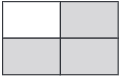2.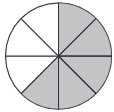3.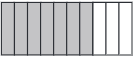4.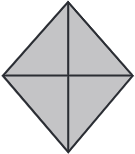11. Express as a mixed fraction:

1. $\frac{101}{4}$
2. $\frac{81}{6}$
12.  Arrange the following in ascending order:
$\frac{2}{15},\frac{1}{15},\frac{6}{15},\frac{12}{15},\frac{7}{15}$

13. Write the next three equivalent to each of the following:

1. $\frac{2}{4}$, __, __, __
2. $\frac{4}{5}$, __, __, __
3. $\frac{1}{3}$, __, __, __

1. Savan completed $\frac{4}{5}$ of 50 pages of his book. how many pages of a book he completed?
2. Arjun is allowed to play cricket for $\frac{8}{3}$ hours each day. he has already played for $\frac{4}{3}$ hours today. what fraction of an hour does Arjun has left to play cricket?
15. Identify the type of the following fractions.

$\frac{5}{11}$,$\frac{15}{11}$$\frac{1}{5}$$1\frac{1}{5}$

16. Multiply:

1. 7$×$$\frac{2}{11}$
2. $\frac{17}{11}$$×$4
3. $4\frac{5}{6}$$×$$1\frac{5}{29}$
4. $\frac{8}{10}$$×$$\frac{1}{10}$

CBSE Worksheet-01
Class - IV Mathematics – Fractional Numbers

Solution

1. Fill in the blanks with suitable words:

1. $\frac{9}{4}$
$\frac{3}{4}$ + __ = $\frac{12}{4}$
__ = $\frac{12}{4}$-$\frac{3}{4}$
__ = $\frac{12-3}{4}$ (by taking LCM)
__ = $\frac{9}{4}$

2. $\frac{47}{10}$
$4\frac{7}{10}$ = $\frac{\left(4×10\right)+7}{10}$ = $\frac{40+7}{10}$  = $\frac{47}{10}$

3. $\frac{21}{5}$
$4\frac{1}{5}$ = $\frac{\left(4×5\right)+1}{5}$ = $\frac{20+1}{5}$  = $\frac{21}{5}$

4. 10
$4\frac{3}{5}+5\frac{2}{5}=$ $\frac{23}{5}+\frac{27}{5}=$ $\frac{23+27}{5}=$$\frac{50}{5}$ =$\frac{10×5}{5}$ = 10

5. 23, In $\frac{a}{b}$ a is numerator and b is denominator.

2. State whether each of the following statement is True or False:

1. True
Total parts = 12
colored parts = 7
Fraction = $\frac{7}{12}$

2. True
$4\frac{1}{5}=\frac{\left(4×5\right)+1}{5}=\frac{21}{5}$

3. True
The denominator of both fractions is 5 so they are like fractions.

4. False
$\frac{1}{2}+\frac{3}{2}+\frac{5}{2}=\frac{9}{2}$

5. True
$\frac{5}{2}-\frac{3}{2}-\frac{2}{2}=\frac{5-3-2}{2}=0$

3. (d) $\frac{1}{2}$
Explanation: Whole part= 4 and colour part = 2
Part of the whole is coloured = $\frac{2}{4}$$\frac{1}{2}$
4. (b) 5
Explanation: The top number in a fraction is numerator of fraction. Numerator in the fraction $\frac{5}{7}$ is 5.
5. (d) $\frac{8}{10}$
Explanation:
$\frac{1}{10}+\frac{3}{10}\phantom{\rule{thinmathspace}{0ex}}\phantom{\rule{thinmathspace}{0ex}}+\phantom{\rule{thinmathspace}{0ex}}\phantom{\rule{thinmathspace}{0ex}}\frac{4}{10}=\frac{1+3+4}{10}=\frac{8}{10}$
6. (b) <
Explanation: Take L.C.M 8,9 =72

$\frac{4}{8}=\frac{4×9}{8×9}=\frac{36}{72}$

$\frac{8}{9}\phantom{\rule{thinmathspace}{0ex}}=\frac{8×8}{9×8}=\frac{64}{72}\phantom{\rule{thinmathspace}{0ex}}$
$\frac{4}{8}\phantom{\rule{thinmathspace}{0ex}}<\frac{8}{9}$

7. (b) $3\frac{1}{5}$

Explanation: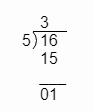$⇒$$\frac{16}{5}=3\frac{1}{5}$

8. (a) – (ii), (b) – (iii), (c) – (i), (d) – (iv)
(a) $\frac{1}{10}.......\frac{4}{10}$
1 < 4  => $\frac{1}{10}<\frac{4}{10}$

(b) $\frac{3}{20}.......\frac{1}{20}$
3 > 1 ==>  $\frac{3}{20}>\frac{1}{20}$

(c) $\frac{2}{10}.......\frac{1}{5}$

$\frac{2}{10}=\frac{1}{5}$

(d) $\frac{2}{10}.......\frac{1}{10}=\frac{3}{10}$
$\frac{2}{10}+\frac{1}{10}=\frac{3}{10}$

9. $\frac{8}{19}$